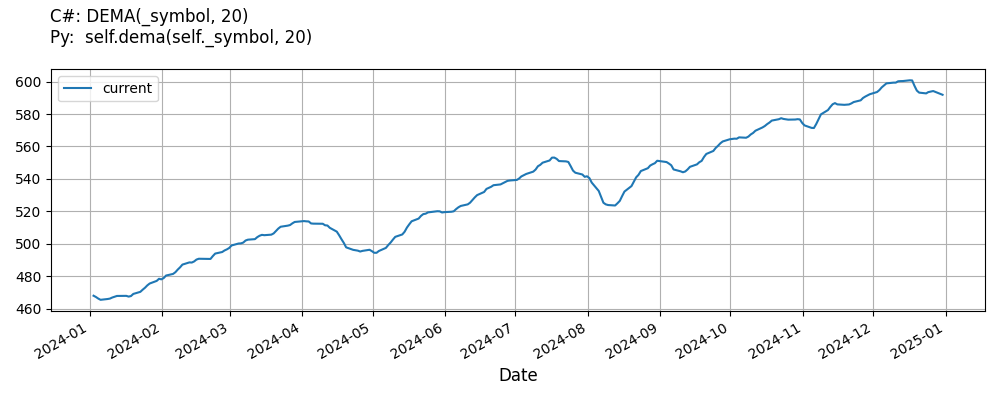# Supported Indicators

## Double Exponential Moving Average

### Introduction

This indicator computes the Double Exponential Moving Average (DEMA). The Double Exponential Moving Average is calculated with the following formula: EMA2 = EMA(EMA(t,period),period) DEMA = 2 * EMA(t,period) - EMA2 The Generalized DEMA (GD) is calculated with the following formula: GD = (volumeFactor+1) * EMA(t,period) - volumeFactor * EMA2

To view the implementation of this indicator, see the LEAN GitHub repository.

### Using DEMA Indicator

To create an automatic indicators for DoubleExponentialMovingAverage, call the DEMA helper method from the QCAlgorithm class. The DEMA method creates a DoubleExponentialMovingAverage object, hooks it up for automatic updates, and returns it so you can used it in your algorithm. In most cases, you should call the helper method in the Initialize method.

public class DoubleExponentialMovingAverageAlgorithm : QCAlgorithm
{
private Symbol _symbol;
private DoubleExponentialMovingAverage _dema;

public override void Initialize()
{
_dema = DEMA(_symbol, 20);
}

public override void OnData(Slice data)
{
{
// The current value of _dema is represented by itself (_dema)
// or _dema.Current.Value
Plot("DoubleExponentialMovingAverage", "dema", _dema);

}
}
}
class DoubleExponentialMovingAverageAlgorithm(QCAlgorithm):
def Initialize(self) -> None:
self.dema = self.DEMA(self.symbol, 20)

def OnData(self, slice: Slice) -> None:
# The current value of self.dema is represented by self.dema.Current.Value
self.Plot("DoubleExponentialMovingAverage", "dema", self.dema.Current.Value)



The following reference table describes the DEMA method:

### DEMA()1/1

            DoubleExponentialMovingAverage QuantConnect.Algorithm.QCAlgorithm.DEMA (
Symbol                           symbol,
Int32                            period,
*Nullable<Resolution>      resolution,
*Func<IBaseData, Decimal>  selector
)


Creates a new DoubleExponentialMovingAverage indicator.

If you don't provide a resolution, it defaults to the security resolution. If you provide a resolution, it must be greater than or equal to the resolution of the security. For instance, if you subscribe to hourly data for a security, you should update its indicator with data that spans 1 hour or longer.

You can manually create a DoubleExponentialMovingAverage indicator, so it doesn't automatically update. Manual indicators let you update their values with any data you choose.

Updating your indicator manually enables you to control when the indicator is updated and what data you use to update it. To manually update the indicator, call the Update method with time/number pair, or an IndicatorDataPoint. The indicator will only be ready after you prime it with enough data.

public class DoubleExponentialMovingAverageAlgorithm : QCAlgorithm
{
private Symbol _symbol;
private DoubleExponentialMovingAverage _dema;

public override void Initialize()
{
_dema = new DoubleExponentialMovingAverage(20);
}

public override void OnData(Slice data)
{
if (data.Bars.TryGeValue(_symbol, out var bar))
{
_dema.Update(bar.EndTime, bar.Close);
}

{
// The current value of _dema is represented by itself (_dema)
// or _dema.Current.Value
Plot("DoubleExponentialMovingAverage", "dema", _dema);

}
}
}
class DoubleExponentialMovingAverageAlgorithm(QCAlgorithm):
def Initialize(self) -> None:
self.dema = DoubleExponentialMovingAverage(20)

def OnData(self, slice: Slice) -> None:
bar = slice.Bars.get(self.symbol)
if bar:
self.dema.Update(bar.EndTime, bar.Close)

# The current value of self.dema is represented by self.dema.Current.Value
self.Plot("DoubleExponentialMovingAverage", "dema", self.dema.Current.Value)



To register a manual indicator for automatic updates with the security data, call the RegisterIndicator method.

public class DoubleExponentialMovingAverageAlgorithm : QCAlgorithm
{
private Symbol _symbol;
private DoubleExponentialMovingAverage _dema;

public override void Initialize()
{
_dema = new DoubleExponentialMovingAverage(20);
RegisterIndicator(_symbol, _dema, Resolution.Daily);
}

public override void OnData(Slice data)
{
{
// The current value of _dema is represented by itself (_dema)
// or _dema.Current.Value
Plot("DoubleExponentialMovingAverage", "dema", _dema);

}
}
}
class DoubleExponentialMovingAverageAlgorithm(QCAlgorithm):
def Initialize(self) -> None:
self.dema = DoubleExponentialMovingAverage(20)
self.RegisterIndicator(self.symbol, self.dema, Resolution.Daily)

def OnData(self, slice: Slice) -> None:
# The current value of self.dema is represented by self.dema.Current.Value
self.Plot("DoubleExponentialMovingAverage", "dema", self.dema.Current.Value)



The following reference table describes the DoubleExponentialMovingAverage constructor:

### DoubleExponentialMovingAverage()1/2

            DoubleExponentialMovingAverage QuantConnect.Indicators.DoubleExponentialMovingAverage (
string    name,
int       period,
*decimal  volumeFactor
)


Initializes a new instance of the DoubleExponentialMovingAverage class using the specified name and period.

### DoubleExponentialMovingAverage()2/2

            DoubleExponentialMovingAverage QuantConnect.Indicators.DoubleExponentialMovingAverage (
int       period,
*decimal  volumeFactor
)


Initializes a new instance of the DoubleExponentialMovingAverage class using the specified period.

### Visualization

The following image shows plot values of selected properties of DoubleExponentialMovingAverage using the plotly library.You can also see our Videos. You can also get in touch with us via Discord.